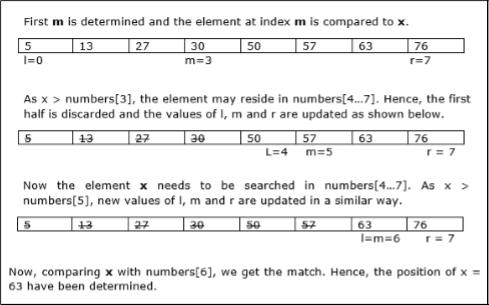• Design and Analysis of Algorithms
• Home

Design and Analysis Binary Search

In this chapter, we will discuss another algorithm based on divide and conquer method.

Problem Statement

Binary search can be performed on a sorted array. In this approach, the index of an element x is determined if the element belongs to the list of elements. If the array is unsorted, linear search is used to determine the position.

Solution

In this algorithm, we want to find whether element x belongs to a set of numbers stored in an array numbers[]. Where l and r represent the left and right index of a sub-array in which searching operation should be performed.

Algorithm: Binary-Search(numbers[], x, l, r)
if l = r then
return l
else
m := ⌊(l + r) / 2⌋
if x ≤ numbers[m]  then
return Binary-Search(numbers[], x, l, m)
else
return Binary-Search(numbers[], x, m+1, r)

Analysis

Linear search runs in O(n) time. Whereas binary search produces the result in O(log n) time

Let T(n) be the number of comparisons in worst-case in an array of n elements.

Hence,

$$T(n)=\begin{cases}0 & if\:n= 1\\T(\frac{n}{2})+1 & otherwise\end{cases}$$

Using this recurrence relation $T(n) = log\:n$.

Therefore, binary search uses $O(log\:n)$ time.

Example

In this example, we are going to search element 63.Useful Video Courses

Video

Compiler Design Online Training

102 Lectures 10 hours

Video

Sequential Circuit Design

30 Lectures 3 hours

Video

Design Patterns Online Training

31 Lectures 4 hours

Video

InDesign Online Training

43 Lectures 1.5 hours

Video

Canva: Become a Graphic Designer

7 Lectures 1 hours

Video

The Ultimate Canva Graphic Design Course

54 Lectures 4 hours